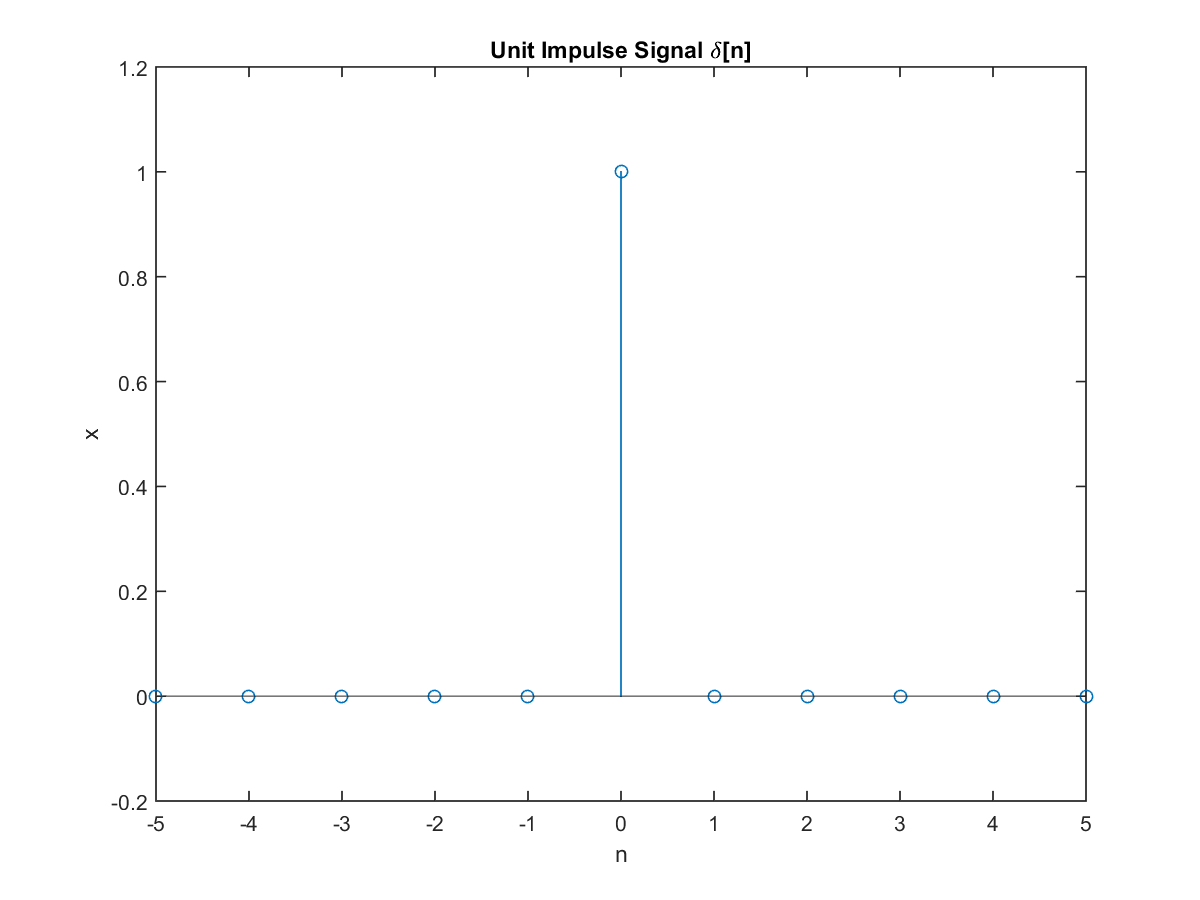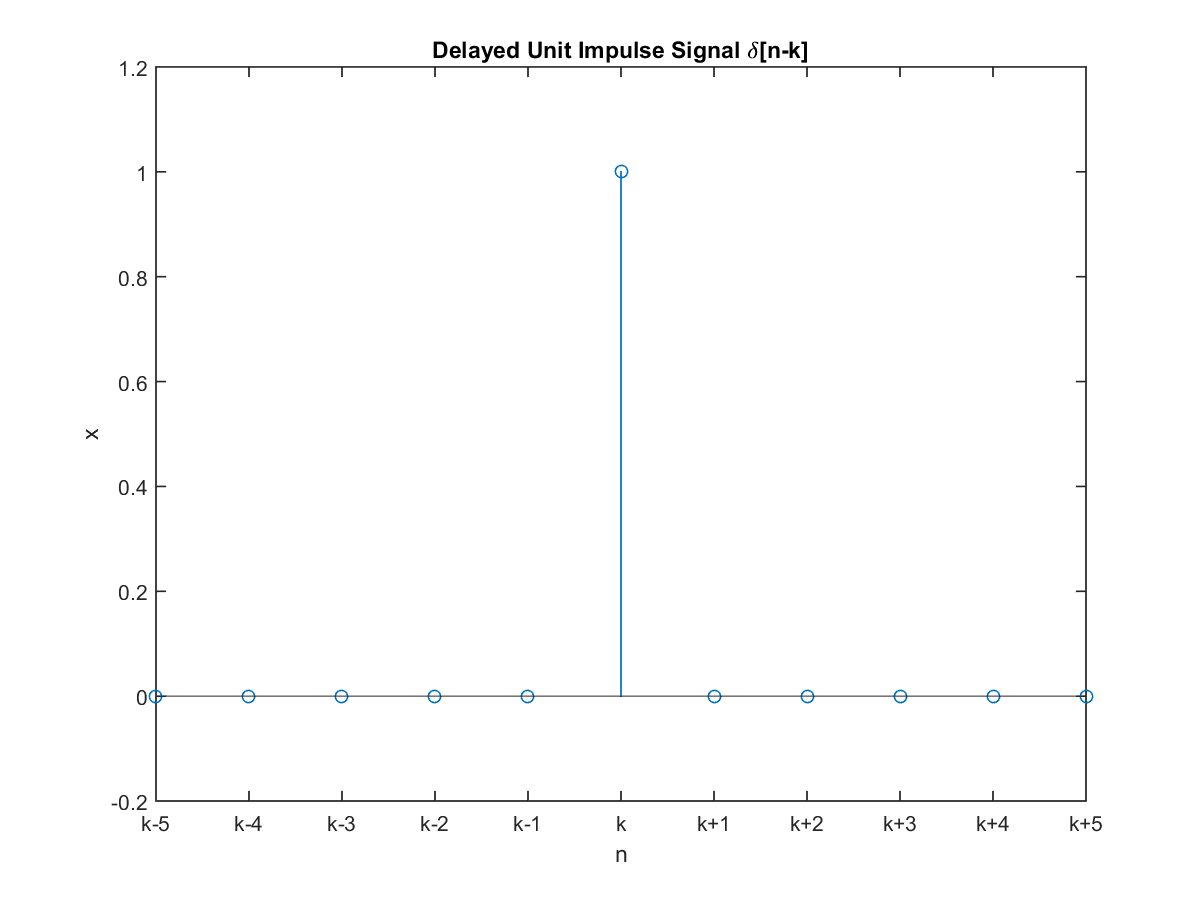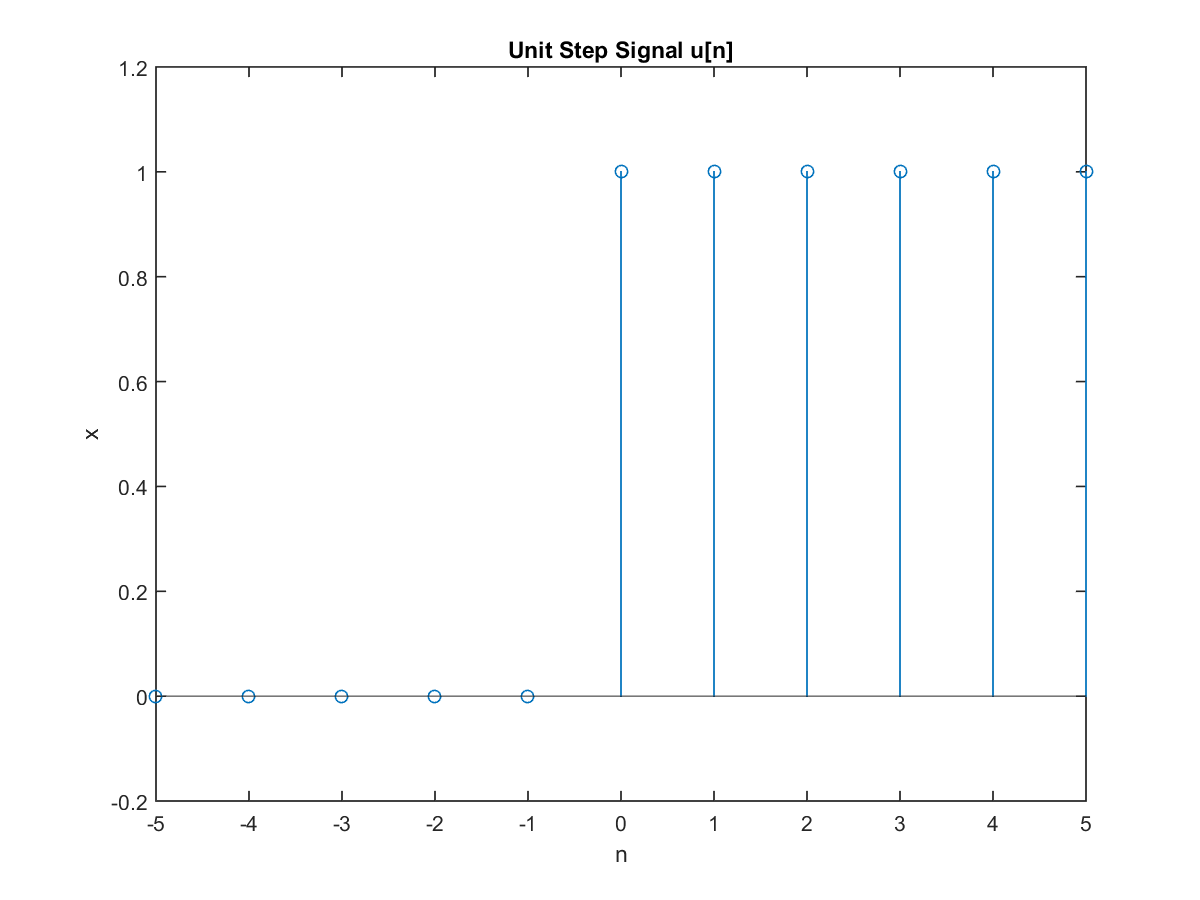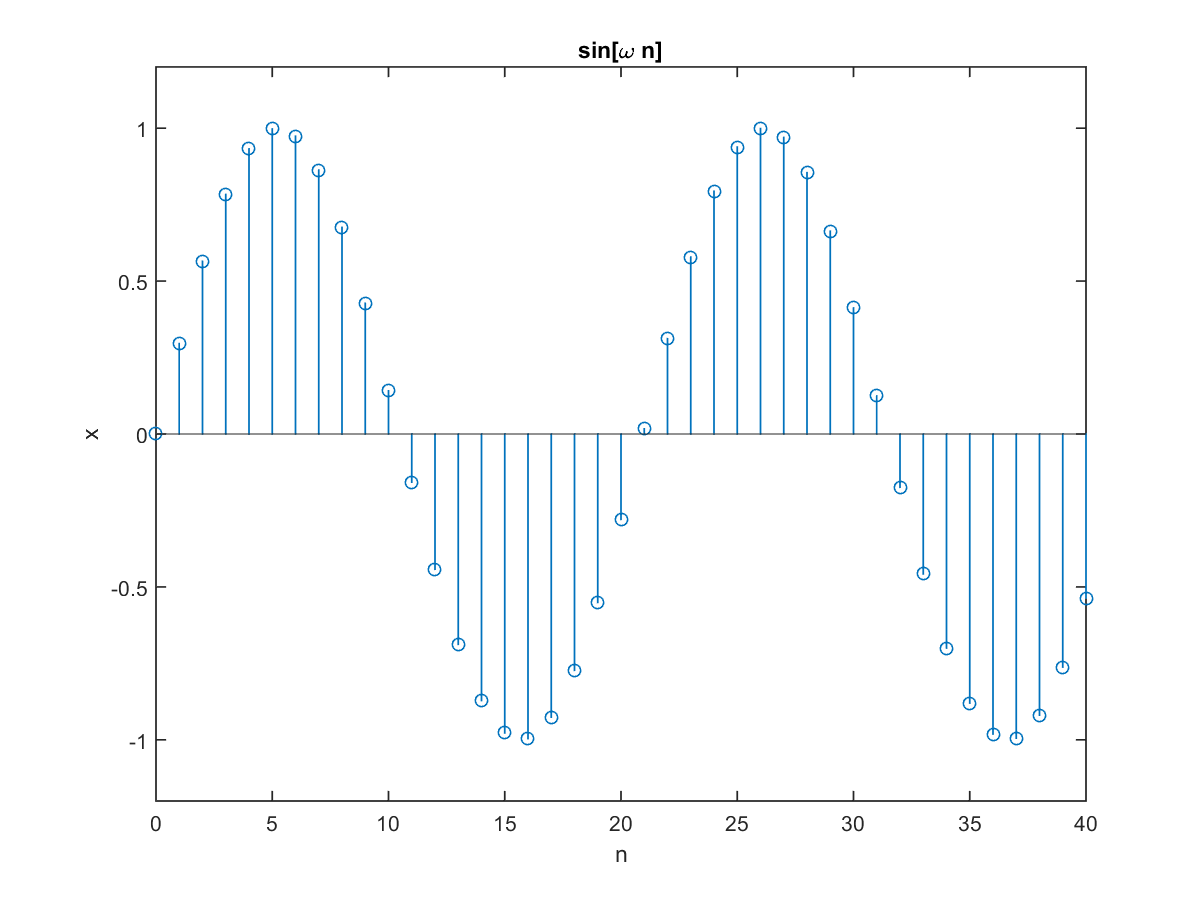## 9-1 Discrete-Time Signals (嚙踝蕭嚙踝蕭嚙踝蕭嚙踝蕭嚙踝蕭嚙踝蕭嚙踝蕭嚙踝蕭嚙踝蕭)

All signals in the real-world are continuous. In contrast, all signals stored in a computer use the unit of byte as the basic storage unit. Therefore these signals are referred to discrete-time signals. In mathematical notations, discrete-time signals can be represented by x(nT), where T is the sampling period (the reciprocal of the sample rate), n is an integer and nT is the elapsed time. For simplicity, we shall use x[n] to represent the discrete-time signal at time nT, with T implicitly defined.

Hint
In fact, signals stored in a computer are discrete in both time and their values. For the discussion for this chapter, we are only concerned with the discretization in time.

In the following, we shall introduce some of the commonly used discrete-time signals:

The unit impulse signal d[n] is zero everywhere except at n = 0, where its value is 1. Mathematically, we can express the unit impulse signal as the following expression:
 d[n] = 1, if n=0 d[n] = 0, otherwise
We can plot the unit impluse signal as follows:

Example 1: impulse01.m% Plot an unit impulse signal n = -5:5; x = 0*n; index=find(n==0); x(index)=1; % plot stem(n, x); axis([-inf, inf, -0.2, 1.2]); xlabel('n'); ylabel('x'); title('Unit Impulse Signal \delta[n]');If we delay the unit impulse signal by k sample points, we have the following equation:
 d[n-k] = 1, if n=k d[n-k] = 0, otherwise
The above delayed unit imulse signal can be plotted as follows:

Example 2: impulse02.m% Plot an unit impulse signal n = -5:5; x = 0*n; index=find(n==0); x(index)=1; % plot stem(n, x); axis([-inf, inf, -0.2, 1.2]); xlabel('n'); ylabel('x'); title('Delayed Unit Impulse Signal \delta[n-k]'); set(gca, 'xticklabel', {'k-5', 'k-4', 'k-3', 'k-2', 'k-1', 'k', 'k+1', 'k+2', 'k+3', 'k+4', 'k+5'});The above delayed unit impulse signal have the property of masking out other signals not at n = k. In other words, if we multiply any signals x[n] (n = -∞∼∞) with d[n-k], only the term of x[n-k] is left. This can be expressed as the following equation:
 x[k] = Sn=-∞∞d[n-k]•x[n]
By using this property, we can express any discrete-time signals as a linear combination of the unit impulse signals. This is especially handy when we derive the response of a linear time-invariant system. See the following section for more details.

The unit step signal u[n] is 1 when n≧0, and 0 elsewhere. In mathematical notations, we have
 u[n] = 1, if n≧0 u[n] = 0, otherwise
The unit step signal can be plotted, as shown in the next example:

Example 3: unitStep01.m% Plot an unit impulse signal n = -5:5; x = 0*n; index=find(n>=0); x(index)=1; % plot stem(n, x); axis([-inf, inf, -0.2, 1.2]); xlabel('n'); ylabel('x'); title('Unit Step Signal u[n]');It should be noted that the unit impulse signal is the first-order difference of the unit step signal:
d[n] = u[n] - u[n-1].
The sinusoidal signal is another commonly used discrete-time signals:
sin[n] = sin(2pf(nT)) = sin(wn))
where f is the oscillation frequency of the sinusoidal signal and w ( = 2pfT) is the normalized angular frequency. The sinusoidal signal can be plotted as follows:

Example 4: sinusoid01.m% Plot a sinusoidal signal n = 0:40; omega=0.3; x = sin(omega*n); % plot stem(n, x); axis([-inf, inf, -1.2, 1.2]); xlabel('n'); ylabel('x'); title('sin[\omega n]');Audio Signal Processing and Recognition (音訊處理與辨識)